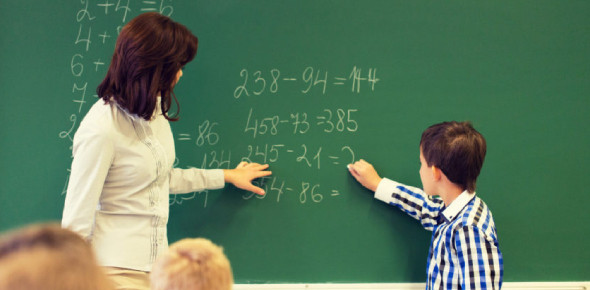# Test How Much You Can Score In 4th Grade Math Quiz?

15 Questions | Total Attempts: 327SettingsSchool can be a little hard, and some people find math to be a particularly hard subject. One way to make this a little easy is ensuring you get in some practice on what you have learned. Can you answer all these questions asked here in the 4th-grade math? Why don’t you try it up and see if you have what it takes?

• 1.
Which number is largest?
• A.

149

• B.

822

• C.

324

• D.

287

• 2.
What is the best estimate for 5679 + 4600?
• A.

10400

• B.

10600

• C.

10300

• D.

10500

• 3.
What is the sum of 51 + 46?
• A.

5

• B.

97

• C.

87

• D.

95

• 4.
What is the best estimate for 661 - 268?
• A.

500

• B.

400

• C.

600

• D.

300

• 5.
What is 3/8 + 1/8?
• A.

Mar-64

• B.

8-Apr

• C.

16-Apr

• D.

4-Mar

• 6.
What is the largest 4 digit number you can write?
• A.

9,999

• B.

999

• C.

9998

• D.

9997

• 7.
What is the best estimate for 27 divided by 4?
• A.

5

• B.

6

• C.

7

• D.

9

• 8.
What is 8.3 + 0.25 + 4.1?
• A.

12.4

• B.

12.65

• C.

14.9

• D.

12.35

• 9.
What is 4217 + 3682 + 5002?
• A.

10911

• B.

12901

• C.

14821

• D.

16889

• 10.
How do you write 10/4 as a mixed numeral in lowest terms?
• A.

10-Apr

• B.

2 1/2

• C.

2 1/2

• D.

2 1/2

• 11.
What is 7285 - 1967?
• A.

5319

• B.

5317

• C.

5328

• D.

5318

• 12.
What is 3/4 + 1/4 written in lowest terms?
• A.

1

• B.

2-Jan

• C.

4-Apr

• D.

8-Apr

• 13.
What is 700 x 6?
• A.

42

• B.

420

• C.

42000

• D.

4200

• 14.
What is 3668 rounded to the nearest thousand?
• A.

3000

• B.

3700

• C.

3600

• D.

4000

• 15.
How do you write the simplified version of 2/8?
• A.

8-Jan

• B.

2-Aug

• C.

4-Jan

• D.

2-Jan

Related TopicsBack to top